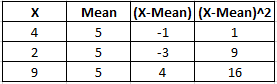## Sample Standard Deviation

Calculate the standard deviation for the sample values: 4, 2, 9

Hint
Sample standard deviation:
$$s =\sqrt{\frac{1}{n-1}\sum_{i=1}^{n} (X_{1}-\mu )^{2}}$$$where $$n$$ is the number of items or observations, $$X$$ is the value from the set, and $$\mu$$ is the mean. Hint 2 We can set up the following table:First, let's find the mean of all the values: $$(4+2+9)/3=5$$ We can set up the following table:Because these are sample values, the standard deviation formula is: $$s =\sqrt{\frac{1}{n-1}\sum_{i=1}^{n} (X_{1}-\mu )^{2}}$$$
where $$n$$ is the number of items or observations, $$X$$ is the value from the set, and $$\mu$$ is the mean. Do not confuse this with a population standard deviation. Plugging the values from the table:
$$\sigma =\sqrt{\frac{(1+9+16)}{3-1}}=\sqrt{\frac{26}{2}}=3.6$$\$
3.6